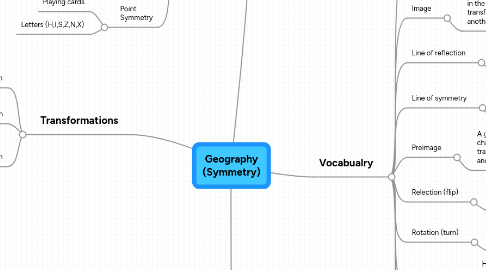# Geography (Symmetry)

Get Started. It's FreeGeography (Symmetry)## 1. Symmetry

### 1.1. Reflection Symmetry

1.1.1. Mirror Symmetry

1.1.2. Line Symmetry

1.1.3. Examples

1.1.3.1. The mountians reflection in water

1.1.3.2. somethings reflection in a mirror

### 1.2. Rotational Symmetry

1.2.1. Examples

1.2.1.1. Dart board (order 10)

1.2.1.2. US bronze star medal (order 5)

1.2.1.3. Propeller blades (depending on how many blades it has is the order it is)

### 1.3. Point Symmetry

1.3.1. Playing cards

1.3.2. Letters (H,I,S,Z,N,X)

## 2. Transformations

### 2.1. Rotation

2.1.1. Turning around the center

### 2.2. Reflection

2.2.1. Flip from one side to the other

### 2.3. Translation

2.3.1. Slide

2.3.1.1. Same distance

2.3.1.2. Same directon

## 4. Vocabualry

### 4.1. Frieze patteren

4.1.1. A geometirc design in a long strip in which an element is repeated over and over. The element may be rotated, translated, and reflected. Frieze patterens are often found on the walls of buildings, on the borders of rugs and tiled floors, and on clothing.

### 4.2. Image

4.2.1. The reflection of and object that you see when you look in the mirror. Also a figure that is produced by a transformation (reflection, translation, or rotation) of another figure.

### 4.3. Line of reflection

4.3.1. A line halfway between a figure (preimage) and its reflected image. In a reflection, a fugure is flipped over the like of reflection.

### 4.4. Line of symmetry

4.4.1. A line drawn through a figure that divides the figure into two parts that are mirror images of each other. The two parts look alike, but face in opposite diretions

### 4.5. Preimage

4.5.1. A geometric figure that is somehow changed (by a reflection, a rotation, or a translation, for example) to produce another figure.

### 4.6. Relection (flip)

4.6.1. the "flipping" of a fugure over a line (the line of reflection) so that its image is the mirror image of the original (preimage)

### 4.7. Rotation (turn)

4.7.1. A movement of a figure around a fixed point, or axis: a turn

### 4.8. Symmetric

4.8.1. Having the same size and shape on either side of a line, or looking the same when turned by some amount less then 360 degrees

### 4.9. Transformation

4.9.1. Something done to a geometric figure that produces a new figure. The most common transformation are translations (slides), reflections (flips), and rotations (turns)

### 4.10. Translation

4.10.1. A movement of a figure along a straight line, a "slide." In a translation, each point of the figure slides the same distance in the same direction.